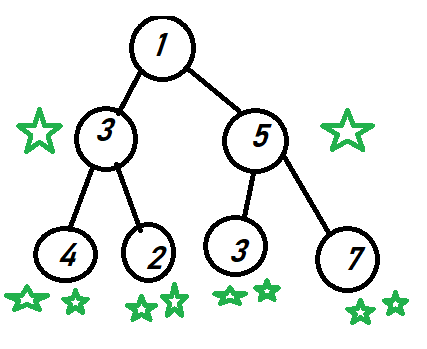# CoDEVIANT #24 — Apples Falling from the Binary TreeYou can visit any apple-tree (node), as often as you like, but you can’t pick up an apple that is already retrieved.
`class BinaryTree {  constructor(value) {    this.value = value;    this.left = null;    this.right = null;  }}function nodeDepths(root) { let depthCount = 0 return helper(root, depthCount) }function helper(node, counter) {  if(!node) {    return 0  }  let numbah = counter +  helper(node.right, counter + 1)  +       helper(node.left, counter + 1)  return numbah}`
`class BinaryTree {  constructor(value) {    this.value = value    this.left = null    this.right = null  }}`
`function nodeDepths(root) {  let depthCount = 0  return helper(root, depthCount)}`
`function helper(node, counter) {  if(!node) {    return 0  }let numbah = counter +  helper(node.right, counter + 1)  +       helper(node.left, counter + 1)  return numbah}`

--

--